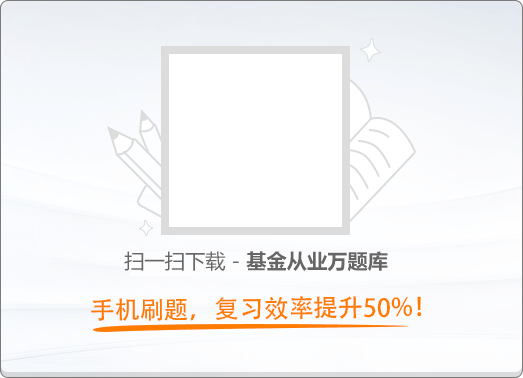120:002016年4月《基金法律法规、职业道德与业务规范》真题（非完整版）

1
(单项选择题)

Ⅰ．风险控制指标标准

Ⅱ．风险评估指标标准

Ⅲ．风险资本准备计算比例

Ⅳ．风险度量方法

• A.

Ⅰ、Ⅱ、Ⅳ

• B.

Ⅰ、Ⅲ

• C.

Ⅰ、Ⅱ

• D.

Ⅱ、Ⅲ、Ⅳ

• A
• B
• C
• D

2
(单项选择题)

• A.

住房按揭交付证券

• B.

银行存款

• C.

经中国证监会认可的国际金融组织发行的证券

• D.

所有的公募基金

• A
• B
• C
• D

3
(单项选择题)

• A.

基金管理人要对每一个重要的风险及其对应的回报进行评价和平衡，采取包括回避、接受、共担或降低这些风险等措施进行风险应对

• B.

事项识别的基础是对事项相关因素进行分析并加以分类，从而识别事项可能带来的风险与机会

• C.

风险评估的过程是根据不同的情况，可采用定性和定量相结合的方法

• D.

员工的风险信息交流意识是风险管理的重要组成部分

• A
• B
• C
• D

4
(单项选择题)

• A.

内部监督

• B.

管理层授权

• C.

信息沟通

• D.

控制环境

• A
• B
• C
• D

5
(单项选择题)

• A.

契约型基金是依据基金管理人、基金托管人之间所签署的基金合同设立的

• B.

基金投资者依法享受权利并承担义务

• C.

基金投资者是基金投资的股东

• D.

基金投资者自取得基金份额后即成为基金份额持有人和基金合同的当事人

• A
• B
• C
• D

6
(单项选择题)

• A.

内部环境中经营理念和经营风格

• B.

行为监控中的特定人员分别管理

• C.

事项识别中的不同管理事项的分类

• D.

控制活动中的不相容职务分离

• A
• B
• C
• D

7
(单项选择题)

• A.

有效性

• B.

健全性

• C.

独立性

• D.

相互制约

• A
• B
• C
• D

8
(单项选择题)

• A.

提高从业人员素质

• B.

促进行业规范发展

• C.

促进同业交流

• D.

对违法违规行为进行行政处罚

• A
• B
• C
• D

9
(单项选择题)

• A.

证券结算风险基金

• B.

保险机构

• C.

中国证监会

• D.

交易所

• A
• B
• C
• D

10
(单项选择题)

《中华人民共和国证券投资基金法》明确规定了基金份额持有人享有的权利，以下关于基金份额持有人的权利，错误的是（　）。

• A.

无需授权即代表全体基金份额持有人利益行使诉讼权利

• B.

对基金管理人、基金托管人、基金服务机构损害其合法权益的行为依法提起诉讼

• C.

对基金份额持有人大会审议事项行使表决权

• D.

参与分配清算后的剩余基金财产

• A
• B
• C
• D

11
(单项选择题)

• A.

基金注册登记机构

• B.

中央结算公司

• C.

基金销售机构

• D.

基金托管人

• A
• B
• C
• D

12
(单项选择题)

• A.

投资者买入某封闭式基金份额将增加该基金份额

• B.

投资者买入封闭式基金份额适用已知价原则

• C.

投资者申购某开放式基金将增加该基金份额

• D.

投资者申购开放式基金份额适用未知价原则

• A
• B
• C
• D

13
(单项选择题)

• A.

金额申购、金额赎回

• B.

金额申购、份额赎回

• C.

份额申购、金额赎回

• D.

份额申购、份额赎回

• A
• B
• C
• D

14
(单项选择题)

• A.

1

• B.

6

• C.

9

• D.

3

• A
• B
• C
• D

15
(单项选择题)

• A.

分级基金

• B.

ETF

• C.

LOF

• D.

社保基金

• A
• B
• C
• D

16
(单项选择题)

• A.

可测原则

• B.

易入原则

• C.

成长原则

• D.

通用原则

• A
• B
• C
• D

17
(单项选择题)

• A.

QDII可以融资购买证券

• B.

QDII可以投资与股权挂钩的结构性投资产品

• C.

QDII可以投资房地产抵押按揭

• D.

QDII可以投资贵金属凭证

• A
• B
• C
• D

18
(单项选择题)

• A.

上市开放式基金

• B.

封闭式基金

• C.

开放式基金

• D.

交易型开放式指数基金

• A
• B
• C
• D

19
(单项选择题)

• A.

继承

• B.

转换

• C.

司法强制执行

• D.

捐赠

• A
• B
• C
• D

20
(单项选择题)

• A.

半年度报告的管理人报告无须披露内部检查报告

• B.

半年度报告要披露过去1个月的净值增长率

• C.

半年度报告只需披露当期的主要会计数据和财务数据

• D.

半年度报告需要进行审计

• A
• B
• C
• D

21
(单项选择题)

• A.

办理非公开募集基金的登记、备案

• B.

对会员与客户之间发生的基金业务纠纷进行调解

• C.

教育和组织会员遵守相关法律及行政法规

• D.

有权对会员进行行政处罚

• A
• B
• C
• D

22
(单项选择题)

Ⅰ．公开发行的股份有限公司股票

Ⅱ．股指期货

Ⅲ．港股通股票

• A.

Ⅱ、Ⅲ

• B.

Ⅰ、Ⅱ、Ⅲ

• C.

Ⅰ、Ⅲ

• D.

Ⅰ、Ⅱ

• A
• B
• C
• D

23
(单项选择题)

QDII基金定期报告中的特殊披露要求有（　）。

Ⅰ．境外投资顾问和境外资产托管人信息

Ⅱ．境外证券投资信息

Ⅲ．外币交易及外币折算相关的信息

Ⅳ．投资顾问主要负责人变动信息

• A.

Ⅰ、Ⅲ、Ⅳ

• B.

Ⅱ、Ⅲ、Ⅳ

• C.

Ⅰ、Ⅱ、Ⅲ

• D.

Ⅲ、Ⅳ、Ⅴ

• A
• B
• C
• D

24
(单项选择题)

• A.

如果某些行为违反了法律，该行为一定是违反道德的

• B.

绝大多数的道德规范都是以法律作为价值基础的

• C.

法律的实施对道德观念的培养具有强化促进作用

• D.

道德在调整范围和约束力两方面均对法律有补充作用

• A
• B
• C
• D

25
(单项选择题)

《证券投资基金法》规定，开放式基金的登记业务（　）。

• A.

只能由基金管理人办理

• B.

只能委托中国证监会认定的第三方独立机构办理

• C.

可以由基金管理人办理，也可以委托中国证监会认定的其他机构办理

• D.

只能由销售机构办理

• A
• B
• C
• D

26
(单项选择题)

• A.

非主要股东为自然人的，在境内外资产管理行业从业10年以上

• B.

非主要股东为自然人的，个人金融资产不低于1000万元人民币

• C.

非主要股东为法人或者其他组织的，净资产不低于3000万元人民币

• D.

非主要股东为法人或者其他组织的，最近5年没有违法记录

• A
• B
• C
• D

27
(单项选择题)

• A.

承销证券

• B.

买卖股票或债券

• C.

向基金管理人、基金托管人出资

• D.

从事承担无限责任的投资

• A
• B
• C
• D

28
(单项选择题)

• A.

基金份额登记机构

• B.

基金销售支付机构

• C.

基金评价机构

• D.

基金投资顾问机构

• A
• B
• C
• D

29
(单项选择题)

• A.

基金份额转换不需要先赎回已持有的基金份额

• B.

基金份额转换费用由基金份额持有人承担

• C.

投资者可以将其持有的基金份额转换为另一基金管理人的另一基金份额

• D.

基金份额转换的效率通常高于先赎回再申购基金份额

• A
• B
• C
• D

30
(单项选择题)

• A.

保守秘密

• B.

守法合规

• C.

忠诚尽责

• D.

诚信守信

• A
• B
• C
• D

31
(单项选择题)

ISDA协议是国际掉期与衍生品协会为国际场外衍生品交易提供的标准协议框架。一方面，交易双方可以减少文件的误解等。另一方面，协议为交易双方提供了一个净额结算制度，即一系列的交易如果互有盈亏，可以抵消按净X型结算。ISDA文件最有助于解决交易中存在的（　）。

• A.

合规风险和操作风险

• B.

合规风险和流动性风险

• C.

法律风险和信用风险

• D.

法律风险和操作风险

• A
• B
• C
• D

32
(单项选择题)

• A.

一个月

• B.

六个月

• C.

十二个月

• D.

三个月

• A
• B
• C
• D

33
(单项选择题)

Ⅰ．经营管理能力

Ⅱ．投资管理能力

Ⅲ．股东背景

Ⅳ．内部控制情况

• A.

Ⅰ、Ⅱ

• B.

Ⅰ、Ⅱ、Ⅲ、Ⅳ

• C.

Ⅰ、Ⅱ、Ⅲ

• D.

Ⅰ、Ⅱ、Ⅳ

• A
• B
• C
• D

34
(单项选择题)

• A.

基金管理公司不得聘用在其它公司管理基金未满1年的基金经理担任投资

• B.

基金经理频繁发生变动的，基金管理公司应当向中国证监会及相关派出机构书面说明情况

• C.

基金经理管理基金未满1年主动提出辞职的，应当严格遵守法律法规和聘用合同竞业禁止有关规定，并向中国证监会及相关派出机构书面说明理由

• D.

基金经理管理基金未满1年的，公司不得变更基金经理。如有特殊情况需要变更的，应当向中国证监会及相关派出机构书面说明理由

• A
• B
• C
• D